# Architecture And Planning - (AR) 2018 GATE Paper (Practice Test)

## 65 Questions MCQ Test GATE Past Year Papers for Practice (All Branches) | Architecture And Planning - (AR) 2018 GATE Paper (Practice Test)

Description
Attempt Architecture And Planning - (AR) 2018 GATE Paper (Practice Test) | 65 questions in 180 minutes | Mock test for GATE preparation | Free important questions MCQ to study GATE Past Year Papers for Practice (All Branches) for GATE Exam | Download free PDF with solutions
QUESTION: 1

Solution:
QUESTION: 2

Solution:
QUESTION: 3

### For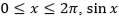and cos �� are both decreasing functions in the interval ________.

Solution:
QUESTION: 4

The area of an equilateral triangle is √3. What is the perimeter of the triangle?

Solution:
QUESTION: 5

Arrange the following three-dimensional objects in the descending order of their volumes:
(i) A cuboid with dimensions 10 cm, 8 cm and 6 cm
(ii) A cube of side 8 cm
(iii) A cylinder with base radius 7 cm and height 7 cm
(iv) A sphere of radius 7 cm

Solution:
QUESTION: 6

An automobile travels from city A to city B and returns to city A by the same route. The speed of the vehicle during the onward and return journeys were constant at 60 km/h and 90 km/h, respectively. What is the average speed in km/h for the entire journey?

Solution:
QUESTION: 7

A set of 4 parallel lines intersect with another set of 5 parallel lines. How many parallelograms are formed?

Solution:
QUESTION: 8

To pass a test, a candidate needs to answer at least 2 out of 3 questions correctly. A total of 6,30,000 candidates appeared for the test. Question A was correctly answered by 3,30,000 candidates. Question B was answered correctly by 2,50,000 candidates. Question C was answered correctly by 2,60,000 candidates. Both questions A and B were answered correctly by 1,00,000 candidates. Both questions B and C were answered correctly by 90,000 candidates. Both questions A and C were answered correctly by 80,000 candidates. If the number of students answering all questions correctly is the same as the number answering none, how many candidates failed to clear the test?

Solution:
QUESTION: 9

If �� 2 + �� − 1 = 0 what is the value of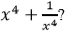Solution:
QUESTION: 10

In a detailed study of annual crow births in India, it was found that there was relatively no growth during the period 2002 to 2004 and a sudden spike from 2004 to 2005. In another unrelated study, it was found that the revenue from cracker sales in India which remained fairly flat from 2002 to 2004, saw a sudden spike in 2005 before declining again in 2006. The solid line in the graph below refers to annual sale of crackers and the dashed line refers to the annual crow births in India. Choose the most appropriate inference from the above data.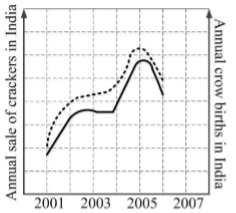Solution:
QUESTION: 11

In a Colour Wheel, Red and Blue colours are

Solution:
QUESTION: 12

In a bird’s eye perspective view of a cuboid, the maximum number of vanishing points is

Solution:
QUESTION: 13

The compressive strength of M-25 concrete is

Solution:
QUESTION: 14

In Critical Path Method (CPM) for time scheduling, ‘forward pass calculation’ is carried out for determining

Solution:
QUESTION: 15

Collapse of the World Trade Center (WTC), New York, in 2001, was due to

Solution:
QUESTION: 16

During the construction of tall buildings, the equipment used for hoisting building materials to the upper floors is a

Solution:
QUESTION: 17

A Rock-cut style of architecture is represented by

Solution:
QUESTION: 18

‘Area based development’ and ‘Pan city development’ are part of

Solution:
QUESTION: 19

In mass transportation, LRTS stands for

Solution:
QUESTION: 20

The structural grid type shown in the figure below is a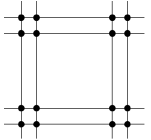Solution:
QUESTION: 21

Assuming other variables remaining constant, the Tropical Summer Index

Solution:
QUESTION: 22

Government of India’s urban development program ‘HRIDAY’ stands for

Solution:
QUESTION: 23

As per the Urban and Regional Development Plan Formulation and Implementation (URDPFI) guidelines, the plan period considered in a ‘Perspective plan’ is

Solution:
QUESTION: 24

The Hall of Nations, New Delhi, was designed by

Solution:
QUESTION: 25

As per the National Building Code of India 2016, the minimum turning radius (in metres) required for fire tender movement is

Solution:
QUESTION: 26

Sidi Bashir Mosque with ‘Shaking Minarets’ is located in

Solution:
QUESTION: 27

‘Sight Distance’ is considered in the design of

Solution:
QUESTION: 28

In India, the term ‘Town Planning Scheme’ refers to

Solution:
QUESTION: 29

Bamboo is a type of

Solution:
QUESTION: 30

According to the UN, one of the components for measuring ‘inclusive growth’ is

Solution:

A (or) B (or) C

QUESTION: 31

The unit of measurement of Damp Proof Course (DPC) in building construction is

Solution:
QUESTION: 32

Which of the following is NOT a Building Information Modeling software tool

Solution:
QUESTION: 33

The concentric circles in a solar chart represent

Solution:
QUESTION: 34

A room of 3m × 3m × 3m has a reverberation time of 0.8 sec. Using Sabine’s method, the total absorption in the room is _____________ sabin (up to one decimal place).

Solution:
QUESTION: 35

A 25 storeyed building has 5 lifts.  The resulting waiting time is 35 sec and ‘Return Travel Time’ is 175 sec.  The number of lifts required for reducing waiting time to 25 sec, without increasing the lift speed, is _______________

Solution:
QUESTION: 36

Match the planning documents in Group-I with their respective government schemes in Group-II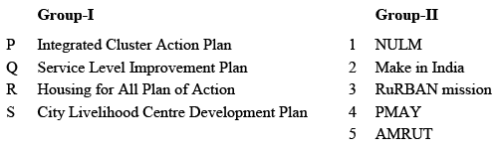Solution:
QUESTION: 37

Associate the fire safety requirements for high rise buildings in Group-I with corresponding standards of the National Building Code of India 2016 in Group-II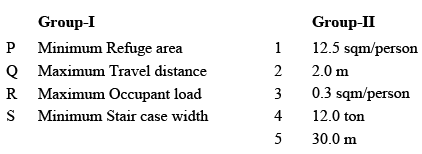Solution:
QUESTION: 38

Match the photometric quantities in Group-I with their respective units in Group-II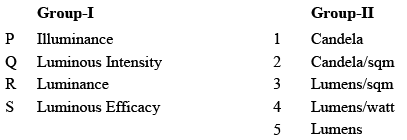Solution:
QUESTION: 39

Associate the symbols in Group I with their meanings in Group II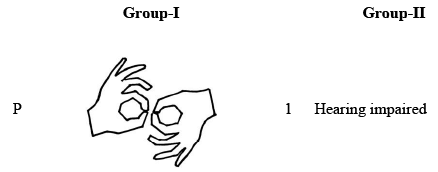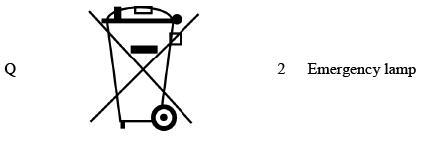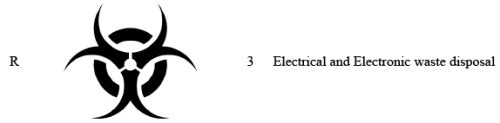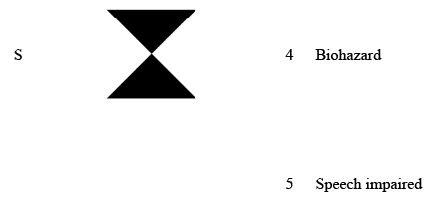Solution:
QUESTION: 40

Match the elements in Group-I with the building components in Group-II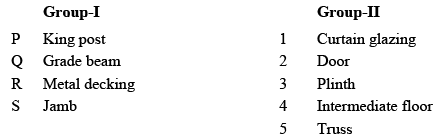Solution:
QUESTION: 41

Match the iconic architectural examples in Group-I with their predominant structural systems in Group-II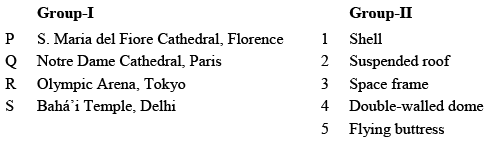Solution:
QUESTION: 42

Match the building materials in Group-I with their distinctive properties in Group-II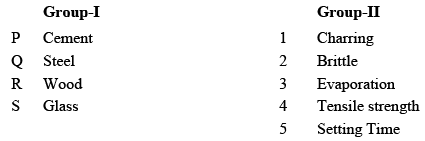Solution:
QUESTION: 43

Match the built forms in Group-I with their descriptions  in Group-II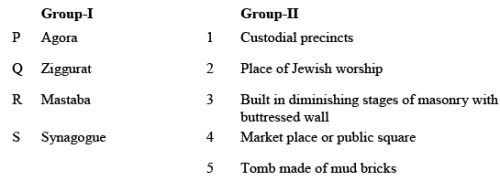Solution:
QUESTION: 44

Match the building configuration characteristics in Group-I with their seismic consequences in Group-II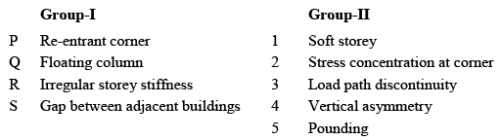Solution:
QUESTION: 45

Match the landscaping terms in Group-I with their descriptions in Group-II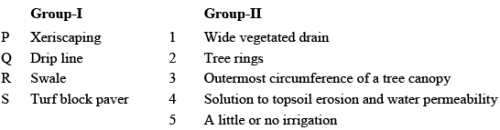Solution:
QUESTION: 46

Match the planning principles in Group-I with their descriptions in Group-II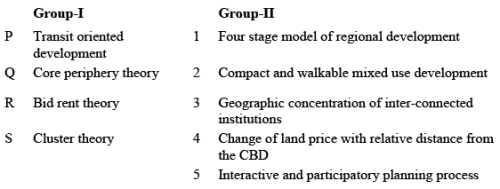Solution:
QUESTION: 47

Match the cities in Group-I with their planners in Group-II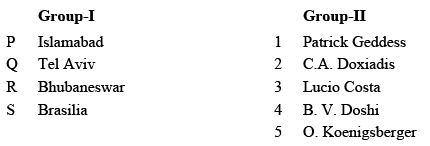Solution:
QUESTION: 48

Match the Temples in Group-I with their Dynastic period in Group-II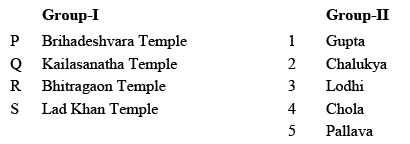Solution:
QUESTION: 49

Match the Buildings in Group-I with their Architects in Group-II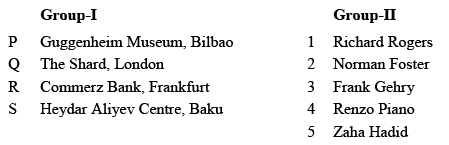Solution:
QUESTION: 50

Match the following urban conservation themes in Group-I with their respective descriptions in Group-II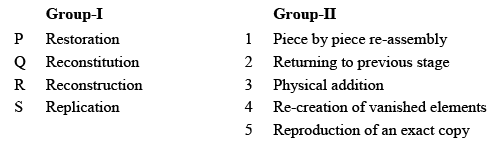Solution:
QUESTION: 51

A Single Phase Neutral (SPN) electrical circuit has a power consumption of 330W. Considering a voltage of 110V and power factor of 0.8, the electrical current drawn is ___________ Amp (up to one decimal place).

Solution:
QUESTION: 52

A building with 100 sqm roof area is connected to a 72 cum rainwater collection tank. If the rainfall is 60 mm per hour and the loss during water storage is 20%, then the time taken to fill the tank completely is_______ hours.

Solution:
QUESTION: 53

The planning norms for provision of schools in a given town is shown in the table below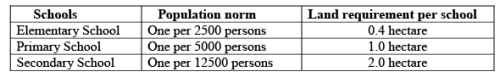Total land area required for providing all types of schools for a population of 200,000 is ___________ hectares.

Solution:
QUESTION: 54

In a mixed use development on a 2.0 hectare site with 2.0 FAR, the ratio of residential to commercial floor area is 3:2. The minimum parking (in ECS) needed per 100 sqm of residential and commercial floor area is 1.0 and 1.25 respectively. Considering full FAR utilization, the total parking requirement is ____________ ECS.

Solution:
QUESTION: 55

A plotted housing scheme on a site of 12 hectare has 60% saleable area. The average unit cost of land development is INR 300 million per hectare. If the profit margin is 20%, then the selling price of land per hectare is __________ million INR.

Solution:
QUESTION: 56

An isolated enclosure shown in the Figure has inlet P and outlet Q of 2 sqm each, on the opposite walls. The outdoor wind speed is 5 m/sec. If the coefficient of effectiveness is 0.6, the rate of natural ventilation in the enclosure due to wind action is _________ cum/hr.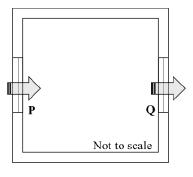Solution:
QUESTION: 57

A 5m × 5m × 3m room has four 230 mm thick external brick walls. Total wall fenestration is 10 sqm. The temperature difference between indoor and outdoor is 2 degC. The air to air transmittance values for 230 mm thick brick wall and 200 mm thick aerated concrete block wall are 2.4 and 1.7 W/sqm degC respectively. If the brick walls are replaced with the aerated concrete block walls, then the change in conductive heat flow through the walls is _________W.

Solution:
QUESTION: 58

For an activity, ‘optimistic time duration’ is 4 days, ‘pessimistic time duration’ is 11 days and ‘most-likely time duration’ is 8 days. The PERT value of time duration is______ days (up to one decimal place).

Solution:
QUESTION: 59

In the Figure, the negative bending moment at point A of the cantilever is ________ kNm.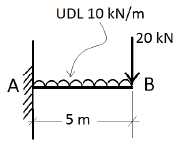Solution:
QUESTION: 60

The water consumption of a high rise apartment building with 60 dwelling units having an average household size of 5 persons is 135 lpcd. Assuming 80% of the total use is met with recycled water supply, the daily domestic demand for the building is _________ litres.

Solution:
QUESTION: 61

In India, for 1.0 cum of M-20 grade concrete, the number of cement bags required is ________ (up to two decimal places).

Solution:
QUESTION: 62

The sound power level of an outdoor non-directional point source is 90 dB. Considering an atmospheric impedance of 400 rayls, the sound pressure level at 10 m distance from the source is _______ dB.

Solution:
QUESTION: 63

The live load and dead load in a three storeyed residential building, transferred through a single column, is 12 tons and 18 tons respectively. If the soil bearing capacity is 10 ton/sqm and the factor of safety is 1.5, the area of column footing is _____________ sqm (up to one decimal place).

Solution:
QUESTION: 64

The indoor illumination requirement for a building is 350 Lux. If the daylight factor is 2.7 and the design sky illuminance is 9000 Lux, then the required supplementary artificial lighting is ____________ Lux.

Solution:
QUESTION: 65

Two design options of a business building on a 10.0 hectare site are being compared for built up area. Floor to floor height of Option A is 3.6 m and that of Option B is 4.5 m. If the maximum allowable building height is 45 m with same ground coverage for both options, the additional built up area achievable in Option A over Option B is______percent.

Solution:Use Code STAYHOME200 and get INR 200 additional OFF Use Coupon Code